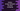# Python numpy reshape() method for array reshaping## Python numpy reshape():

Python numpy reshape() method is used to change the shape of an array without changing the content of the array.

In this post, we will learn how to use reshape() method of numpy with example.

### Definition of numpy.reshape():

This method is defined as below:

``numpy.reshape(array, new_shape, order)``
• array is the array to reshape
• new_shape is the new shape of the array
• order is optional. This is the index order used to read the elements of the array and to place the items in the new shaped array. It can be ‘C’ or ‘F’ or ‘A’. ‘C’ is used for C like index order, F is for fortran like index order and A is for fortran like index order if array is fortran contiguous in memory.

It returns a ndarray, i.e. the new reshaped array.

### Example of reshaping 1-D to 2-D:

The below example shows how to convert a 1-D array to 2-D:

``````import numpy as np

given_array = np.array([1, 2, 3, 4, 5, 6])
new_array = given_array.reshape(3, 2)

print(new_array)``````

It will print the below output:

``````[[1 2]
[3 4]
[5 6]]``````

### Invalid reshaping:

It throws ValueError if the reshape is for invalid values:

``````import numpy as np

given_array = np.array([1, 2, 3, 4, 5, 6])
new_array = given_array.reshape(5, 2)

print(new_array)``````

### Example of reshaping 1-D to 3-D:

Conversion is possible for any dimension. For example, the below script converts a 1-D array to 3-D:

``````import numpy as np

given_array = np.array([1, 2, 3, 4, 5, 6, 7, 8])
new_array = given_array.reshape(2, 2, 2)

print(new_array)``````

It will print:

``````[[[1 2]
[3 4]]
[[5 6]
[7 8]]]``````

### -1 as dimension:

We can also pass -1 as the dimension. numpy will decide what the dimension should be. For example, let’s try to pass -1 as the third dimension for the above example:

``````import numpy as np

given_array = np.array([1, 2, 3, 4, 5, 6, 7, 8])
new_array = given_array.reshape(2, 2, -1)

print(new_array)``````

It will print same output as the above example.

### n-D to 1-D example:

reshape can be used to convert a n-D array to 1-D array. If we pass -1 to reshape(), it flattens the array. For example:

``````import numpy as np

given_array = np.array([[1, 2], [3, 4], [5, 6], [7, 8]])

new_array = given_array.reshape(-1)
print(new_array)``````

It will create the new array new_array, which is a 1-D array. It will print the below output:

``[1 2 3 4 5 6 7 8]``